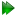The sum of chi-squared values

For the single residue models ofandthe chi-squared value χ2i which is optimised is simply Equation (15.13) on pagein which the relaxation data is that of residue i. However for the global modelsandin which all selected residues are involved the optimised chi-squared value is the sum of those for each residue,

 χ2 =χ2i, (15.8)

where i is the residue index and l is the total number of residues used in the analysis. This is equivalent to Equation (15.13) when the index i ranges over the relaxation data of all selected residues.

The relax user manual (PDF), created 2020-08-26.